Author: Oscar Cronquist Article last updated on October 19, 2022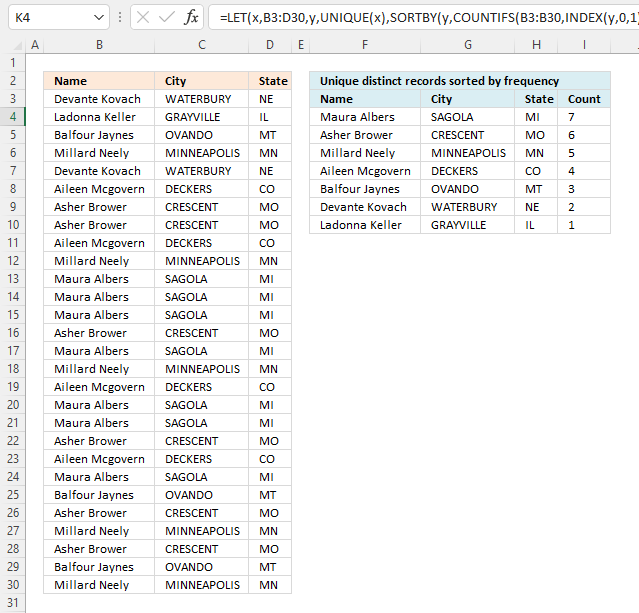This article demonstrates how to sort records in a data set based on their count meaning the formula counts each record and returns a unique distinct list sorted based on a count from large to small.

For example, the first record is shown in cell range F4:H4. The count is in cell I4, it corresponds to the number of times the record is repeated in cell range B3:D30.

This tells us that the record is displayed seven times and is the most repeated record, it is at the very top of the list.

I will also show a much smaller formula that works only in Excel 365, that formula is entered as a regular formula despite the fact that it returns an array of values.

Lastly, a solution for a Pivot Table is also demonstrated.

## 1. Unique distinct records sorted based on count or frequency - Excel 365This formula extracts unique distinct rows in cell range B3:D30 and returns a sorted list based on how many times each row is repeated in B3:D30 from large to small.

Excel 365 formula in cell F4:

=LET(y, UNIQUE(B3:D30), SORTBY(y, COUNTIFS(B3:B30, INDEX(y, 0, 1), C3:C30, INDEX(y, 0, 2), D3:D30, INDEX(y, 0, 3)), -1))

You need to change the formula if the data source has more or fewer columns than this example. A data source containing two columns B3:C30 shrinks the formula to this:

=LET(y, UNIQUE(B3:C30), SORTBY(y, COUNTIFS(B3:B30, INDEX(y, 0, 1), C3:C30, INDEX(y, 0, 2)), -1))

### 2.1 Explaining the formula in cell F4

#### Step 1 - Extract unique distinct records

The UNIQUE function extracts unique distinct values or records.

UNIQUE(array,[by_col],[exactly_once])

UNIQUE(B3:D30)

returns the following data set, see image below.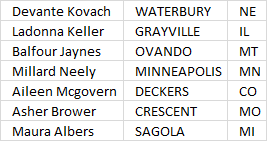#### Step 2 - Extract column from unique distinct records

The INDEX function lets you extract an entire column from an array. This is possible if you use 0 (zero) in the row argument meaning all rows will be extracted. The third argument will lets you choose which column to extract.

Number 1 extracts the first column in the array.

INDEX(array[row_num][column_num], [area_num])

INDEX(UNIQUE(B3:D30),0,1)

returns

{"Devante Kovach"; "Ladonna Keller"; "Balfour Jaynes"; "Millard Neely"; "Aileen Mcgovern"; "Asher Brower"; "Maura Albers"}.

#### Step 3 - Count records

The COUNTIFS function calculates the number of cells across multiple ranges that equals all given conditions.

COUNTIFS(criteria_range1criteria1, [criteria_range2criteria2]…)

COUNTIFS(B3:B30, INDEX(UNIQUE(B3:D30), 0, 1), C3:C30, INDEX(UNIQUE(B3:D30), 0, 2), D3:D30, INDEX(UNIQUE(B3:D30), 0, 3))

returns {2; 1; 3; 5; 4; 6; 7}.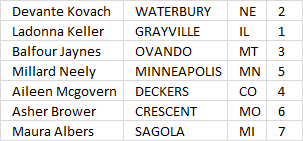The array of numbers is displayed in the image above, each number corresponds to the record on the same row. We can use this relationship to sort the records based on the count.

#### Step 4 - Sort by count

The SORTBY function lets you sort values from a cell range or array based on a corresponding cell range or array. It sorts values by column but keeps rows.

SORTBY(array, by_array1, [sort_order1], [by_array2, sort_order2],…)

SORTBY(UNIQUE(B3:D30), COUNTIFS(B3:B30, INDEX(UNIQUE(B3:D30), 0, 1), C3:C30, INDEX(UNIQUE(B3:D30), 0, 2), D3:D30, INDEX(UNIQUE(B3:D30), 0, 3)), -1)

becomes

SORTBY(UNIQUE(B3:D30), {2; 1; 3; 5; 4; 6; 7}, -1)

and returns the following array: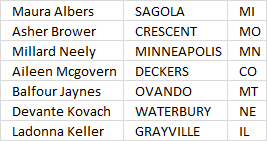The records are now sorted based on the count from large to small.

#### Step 5 - Shorten formula

The formula can be shortened using the LET function. The LET function lets you name intermediate calculation results which can shorten formulas considerably and improve performance.

LET(name1name_value1calculation_or_name2, [name_value2calculation_or_name3...])

SORTBY(UNIQUE(B3:D30), COUNTIFS(B3:B30, INDEX(UNIQUE(B3:D30), 0, 1), C3:C30, INDEX(UNIQUE(B3:D30), 0, 2), D3:D30, INDEX(UNIQUE(B3:D30), 0, 3)), -1)

UNIQUE(B3:D30) is used a lot in the formula.

y - UNIQUE(B3:D30)

LET(y, UNIQUE(B3:D30), SORTBY(y, COUNTIFS(B3:B30, INDEX(y, 0, 1), C3:C30, INDEX(y, 0, 2), D3:D30, INDEX(y, 0, 3)), -1))

## Get Excel *.xlsx file

### Get the Excel fileUnique-distinct-records-sorted-by-frequencyv2.xlsx

## 2. Unique distinct records sorted based on count - Pivot Table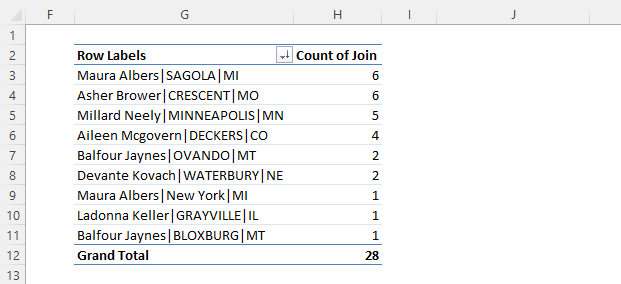This example demonstrates how to count unique distinct records in a Pivot Table. The image above shows a Pivot Table containing nine different records sorted based on the count, here is how I created this Pivot Table calculation.

### 2.1 Add a helper column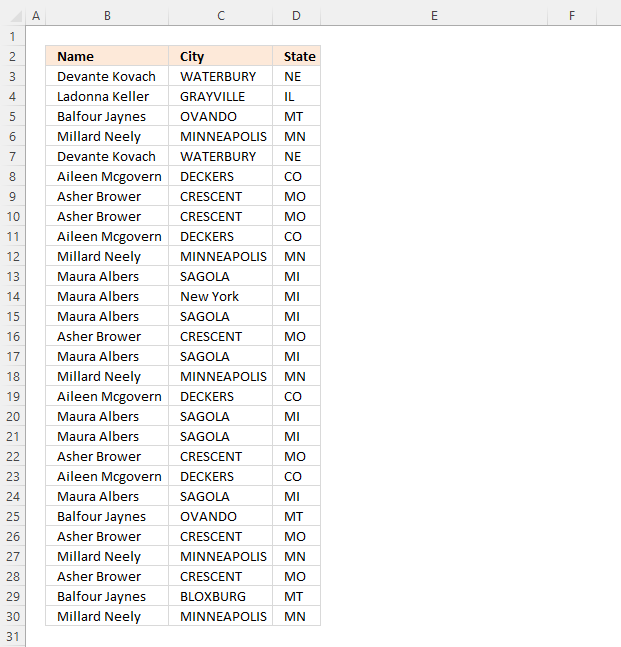The image above shows the source data I am using in this example. The Pivot Table can calculate the count of unique distinct values but not unique distinct records as far as I know.

We need a helper column that merges all values row by row.

Formula in cell E3:

=TEXTJOIN("|",FALSE,B3:D3)

Copy cell E3 and paste to cells below as far as needed.

This formula uses a delimiting character that is not used in the original data source. It is important that you choose a "unique" delimiting character not used in the original data source in order to be able to count unique distinct records.

Here is an example that shows what might happen if we don't choose a "unique" delimiting character. For example, Value "A" and "BB" returns "ABB" if we join the values without a delimiting character.

Value "AB" and "B" also return "ABB", however, the record is different. Let's try this example with a delimiting character "|". "A" and "BB" returns "A|BB" and "AB" and "B" returns ""AB|B".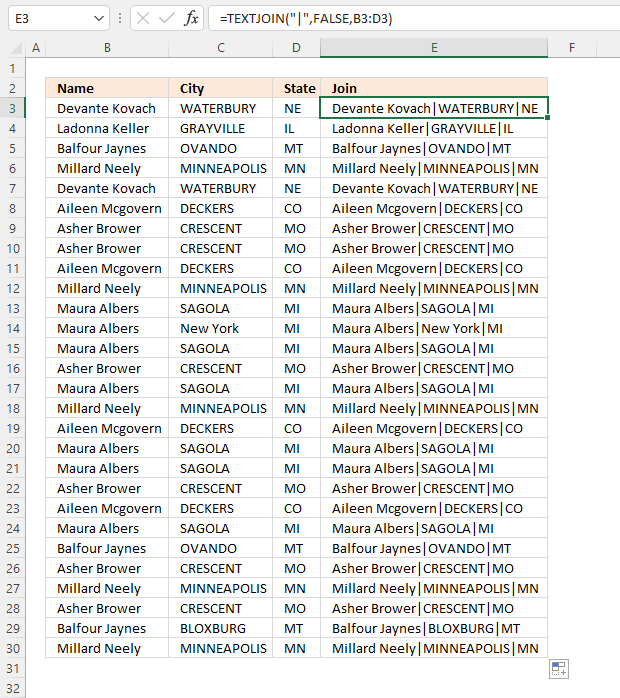### 2.2 Insert a Pivot Table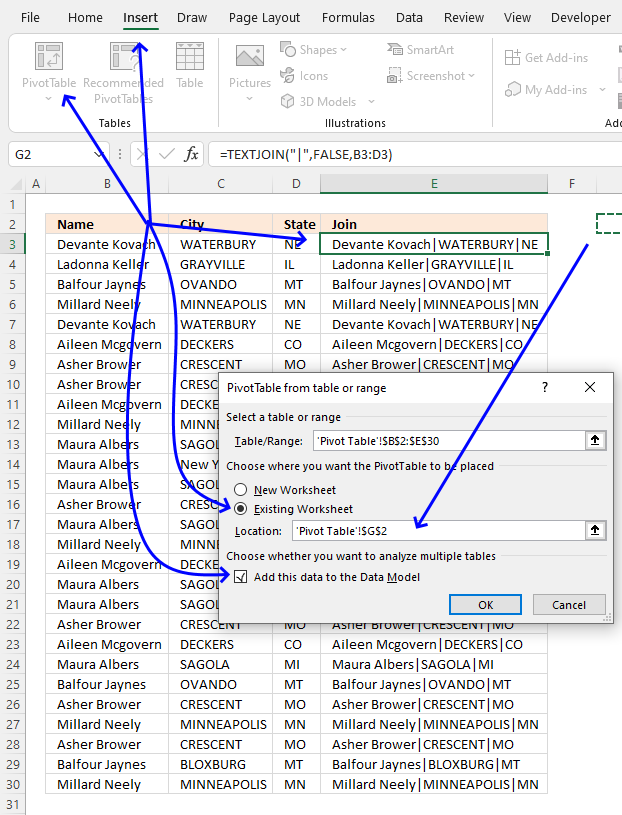1. Select any cell in the data. I chose cell E3, see the image above.
2. Go to tab "Insert" on the ribbon.
3. Press with left mouse button on the "Pivot Table" button.
4. A dialog box appears, select "Existing Worksheet".
5. Select a destination cell by press with left mouse button oning the arrow button. I chose cell G2, see the image above.
6. Press with left mouse button on the check box "Add this data to the Data Model".
7. Press with left mouse button on "OK" button to apply changes.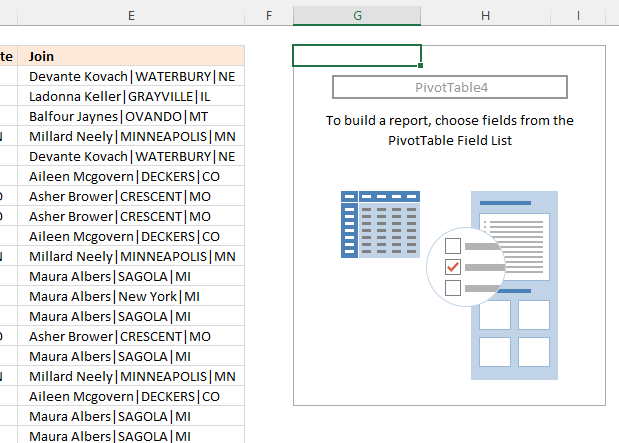An empty pivot table appears in cell G2, see the image above.

### 2.4 Configure Pivot Table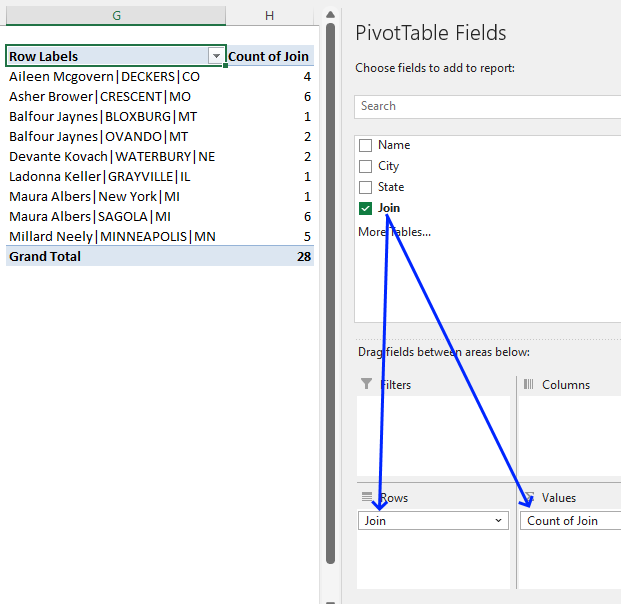1. Select any cell in the empty Pivot Table.
2. A settings pane appears to the right, see the image above.
3. Press and hold with left mouse button on "Join" located in the PivotTable Fields.
4. Drag with mouse to "Rows" field.
5. Repeat step 3 and then drag to the "Values" field, see the image above.

### 2.5 Sort Pivot Table based on the count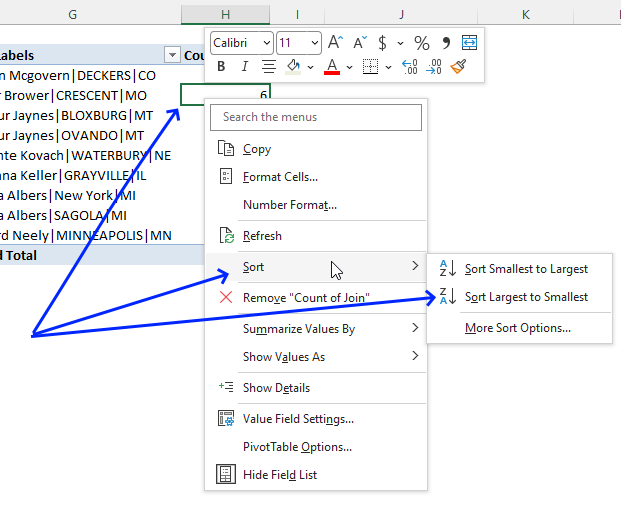1. Press with right mouse button on on any cell in the column "Count of Join".
2. A popup menu appears, press with left mouse button on "Sort".
3. Another popup menu appears, press with left mouse button on "Sort Largest to Smallest".The pivot table now shows unique distinct records sorted by count.

### Get Excel file

Unique distinct records sorted by frequencyv3

## 3. Unique distinct records sorted based on count or frequency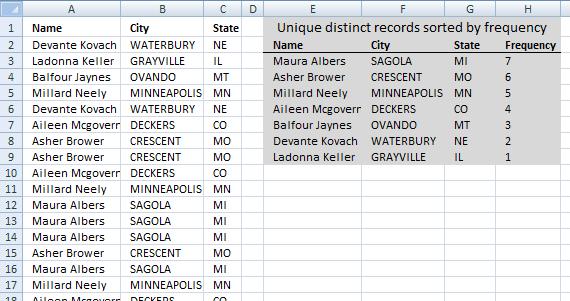How can you use large with multiple criteria?? Example looking for top 5 of a list based on name, state and city..

The formula entered in cell E3 extracts unique distinct records sorted based on frequency or count.

Array formula in cell E3:

=INDEX(\$A\$2:\$C\$29, MATCH(LARGE(IF(COUNTIFS(\$E\$2:E2, \$A\$2:\$A\$29, \$F\$2:F2, \$B\$2:\$B\$29, \$G\$2:G2, \$C\$2:\$C\$29)=0, COUNTIFS(\$A\$2:\$A\$29, \$A\$2:\$A\$29, \$B\$2:\$B\$29, \$B\$2:\$B\$29, \$C\$2:\$C\$29, \$C\$2:\$C\$29), ""), 1), COUNTIFS(\$A\$2:\$A\$29, \$A\$2:\$A\$29, \$B\$2:\$B\$29, \$B\$2:\$B\$29, \$C\$2:\$C\$29, \$C\$2:\$C\$29)*(COUNTIFS(\$E\$2:E2, \$A\$2:\$A\$29, \$F\$2:F2, \$B\$2:\$B\$29, \$G\$2:G2, \$C\$2:\$C\$29)=0), 0), COLUMN(A1))

### 1.1 How to enter an array formula

1. Copy above array formula.
2. Select cell E3
3. Paste array formula to cell E3
4. Press and hold CTRL + SHIFT simultaneously.
5. Press Enter once.
6. Release all keys.

If you did it right the formula is now enclosed with curly brackets, don't enter these characters yourself. They appear automatically if you followed the above steps.

### 1.2 How to copy array formula and paste to remaining cells

1.  Select cell E3
2. Copy cell (not formula). (CTRL + c)
3. Select cell range F3:G3
4. Paste to cell range F3:G3. (CTRL + v)
5. Select cell range E3:G3.
6. Copy cells (not formulas). (CTRL + c)
7. Select cell range E4:G9.
8. Paste to cell range E4:G9. (CTRL + v)

Regular formula in cell H3:

=COUNTIFS(\$A\$2:\$A\$29, E3, \$B\$2:\$B\$29, F3, \$C\$2:\$C\$29, G3)

### 1.3 Explaining array formula in cell E3

#### Step 1 - Identify records not displayed yet

The COUNTIFS function allows you to count cells based on multiple conditions, this makes it ideal for identifying unique distinct records, one condition corresponds to one value in the record.

If you have three values in one record then the COUNTIFS function contains three conditions or six arguments. Each condition has two arguments, the first argument in each pair is a growing cell reference meaning it expands when you copy the cell and paste to cells below.

COUNTIFS(\$E\$2:E2,\$A\$2:\$A\$29,\$F\$2:F2,\$B\$2:\$B\$29,\$G\$2:G2,\$C\$2:\$C\$29)=0

becomes

{0; 0; 0; 0; 0; 0; 0; 0; 0; 0; 0; 0; 0; 0; 0; 0; 0; 0; 0; 0; 0; 0; 0; 0; 0; 0; 0; 0}=0

and returns

{TRUE; TRUE; TRUE; TRUE; TRUE; TRUE; TRUE; TRUE; TRUE; TRUE; TRUE; TRUE; TRUE; TRUE; TRUE; TRUE; TRUE; TRUE; TRUE; TRUE; TRUE; TRUE; TRUE; TRUE; TRUE; TRUE; TRUE; TRUE}.

This makes sense since no values have been displayed yet by the formula in cells above cell E3.

#### Step 2 - Calculate record frequency

The COUNTIFS function also lets you count identical records, by using the IF function we can convert the boolean values to the corresponding count if TRUE and blank "" if FALSE.

IF(COUNTIFS(\$E\$2:E2, \$A\$2:\$A\$29, \$F\$2:F2, \$B\$2:\$B\$29, \$G\$2:G2, \$C\$2:\$C\$29)=0, COUNTIFS(\$A\$2:\$A\$29, \$A\$2:\$A\$29, \$B\$2:\$B\$29, \$B\$2:\$B\$29, \$C\$2:\$C\$29, \$C\$2:\$C\$29), "")

becomes

IF({TRUE; TRUE; TRUE; TRUE; TRUE; TRUE; TRUE; TRUE; TRUE; TRUE; TRUE; TRUE; TRUE; TRUE; TRUE; TRUE; TRUE; TRUE; TRUE; TRUE; TRUE; TRUE; TRUE; TRUE; TRUE; TRUE; TRUE; TRUE}, COUNTIFS(\$A\$2:\$A\$29, \$A\$2:\$A\$29, \$B\$2:\$B\$29, \$B\$2:\$B\$29, \$C\$2:\$C\$29, \$C\$2:\$C\$29), "")

becomes

IF({TRUE; TRUE; TRUE; TRUE; TRUE; TRUE; TRUE; TRUE; TRUE; TRUE; TRUE; TRUE; TRUE; TRUE; TRUE; TRUE; TRUE; TRUE; TRUE; TRUE; TRUE; TRUE; TRUE; TRUE; TRUE; TRUE; TRUE; TRUE}, {2; 1; 3; 5; 2; 4; 6; 6; 4; 5; 7; 7; 7; 6; 7; 5; 4; 7; 7; 6; 4; 7; 3; 6; 5; 6; 3; 5}, "")

and returns

{2; 1; 3; 5; 2; 4; 6; 6; 4; 5; 7; 7; 7; 6; 7; 5; 4; 7; 7; 6; 4; 7; 3; 6; 5; 6; 3; 5}.

The first value in the array above corresponds to the first record (row) in cell range \$A\$2:\$C\$29, thus 2 tells us that there is an identical record somewhere in cell range \$A\$2:\$C\$29. If we examine the list above you can see that the first record (row 2) is identical to the record in row 6.

Row 6 is the fifth value in the array above, that value must also be 2 if the formula works as intended. Yes, the fifth value is also 2.

#### Step 3 - Extract largest number from array

The LARGE function lets you extract the k-th largest value in a cell range or array, in this case, we are looking for the largest value and k is therefore 1.

LARGE(IF(COUNTIFS(\$E\$2:E2, \$A\$2:\$A\$29, \$F\$2:F2, \$B\$2:\$B\$29, \$G\$2:G2, \$C\$2:\$C\$29)=0, COUNTIFS(\$A\$2:\$A\$29, \$A\$2:\$A\$29, \$B\$2:\$B\$29, \$B\$2:\$B\$29, \$C\$2:\$C\$29, \$C\$2:\$C\$29), ""), 1)

becomes

LARGE({2; 1; 3; 5; 2; 4; 6; 6; 4; 5; 7; 7; 7; 6; 7; 5; 4; 7; 7; 6; 4; 7; 3; 6; 5; 6; 3; 5},1)

and returns 7.

#### Step 4 - Find position of largest value

The MATCH function returns the relative position of a value in an array or cell range. The array we match against is the frequency numbers multiplied with an array with boolean values that indicates if a record has already been shown in cells above the current cell.

MATCH(LARGE(IF(COUNTIFS(\$E\$2:E2,\$A\$2:\$A\$29,\$F\$2:F2,\$B\$2:\$B\$29,\$G\$2:G2,\$C\$2:\$C\$29)=0,COUNTIFS(\$A\$2:\$A\$29,\$A\$2:\$A\$29,\$B\$2:\$B\$29,\$B\$2:\$B\$29,\$C\$2:\$C\$29,\$C\$2:\$C\$29),""),1),COUNTIFS(\$A\$2:\$A\$29,\$A\$2:\$A\$29,\$B\$2:\$B\$29,\$B\$2:\$B\$29,\$C\$2:\$C\$29,\$C\$2:\$C\$29)*(COUNTIFS(\$E\$2:E2,\$A\$2:\$A\$29,\$F\$2:F2,\$B\$2:\$B\$29,\$G\$2:G2,\$C\$2:\$C\$29)=0),0)

becomes

MATCH(7,COUNTIFS(\$A\$2:\$A\$29,\$A\$2:\$A\$29,\$B\$2:\$B\$29,\$B\$2:\$B\$29,\$C\$2:\$C\$29,\$C\$2:\$C\$29)*(COUNTIFS(\$E\$2:E2,\$A\$2:\$A\$29,\$F\$2:F2,\$B\$2:\$B\$29,\$G\$2:G2,\$C\$2:\$C\$29)=0),0)

becomes

MATCH(7,{2; 1; 3; 5; 2; 4; 6; 6; 4; 5; 7; 7; 7; 6; 7; 5; 4; 7; 7; 6; 4; 7; 3; 6; 5; 6; 3; 5}*(COUNTIFS(\$E\$2:E2,\$A\$2:\$A\$29,\$F\$2:F2,\$B\$2:\$B\$29,\$G\$2:G2,\$C\$2:\$C\$29)=0),0)

becomes

MATCH(7,{2; 1; 3; 5; 2; 4; 6; 6; 4; 5; 7; 7; 7; 6; 7; 5; 4; 7; 7; 6; 4; 7; 3; 6; 5; 6; 3; 5}*{TRUE; TRUE; TRUE; TRUE; TRUE; TRUE; TRUE; TRUE; TRUE; TRUE; TRUE; TRUE; TRUE; TRUE; TRUE; TRUE; TRUE; TRUE; TRUE; TRUE; TRUE; TRUE; TRUE; TRUE; TRUE; TRUE; TRUE; TRUE},0)

becomes

MATCH(7,{2; 1; 3; 5; 2; 4; 6; 6; 4; 5; 7; 7; 7; 6; 7; 5; 4; 7; 7; 6; 4; 7; 3; 6; 5; 6; 3; 5},0)

and returns 11. Number 7 is found int the 11-th position in the array.

#### Step 5 - Return value based on position

INDEX(\$A\$2:\$C\$29,MATCH(LARGE(IF(COUNTIFS(\$E\$2:E2,\$A\$2:\$A\$29,\$F\$2:F2,\$B\$2:\$B\$29,\$G\$2:G2,\$C\$2:\$C\$29)=0,COUNTIFS(\$A\$2:\$A\$29,\$A\$2:\$A\$29,\$B\$2:\$B\$29,\$B\$2:\$B\$29,\$C\$2:\$C\$29,\$C\$2:\$C\$29),""),1),COUNTIFS(\$A\$2:\$A\$29,\$A\$2:\$A\$29,\$B\$2:\$B\$29,\$B\$2:\$B\$29,\$C\$2:\$C\$29,\$C\$2:\$C\$29)*(COUNTIFS(\$E\$2:E2,\$A\$2:\$A\$29,\$F\$2:F2,\$B\$2:\$B\$29,\$G\$2:G2,\$C\$2:\$C\$29)=0),0),COLUMN(A1))

becomes

INDEX(\$A\$2:\$C\$29,11,COLUMN(A1))

becomes

INDEX(\$A\$2:\$C\$29, 11, 1)

and returns "Maura Albers" in cell E3.